﻿ Inhomogeneous Model for the Investigation of the Optical Properties of the A-Plane Oriented ZnO Epilayers Grown by Plasma-Assisted Molecular Beam Epitaxy

# Journal of Lasers, Optics & PhotonicsOpen Access

All submissions of the EM system will be redirected to Online Manuscript Submission System. Authors are requested to submit articles directly to Online Manuscript Submission System of respective journal.

# Inhomogeneous Model for the Investigation of the Optical Properties of the A-Plane Oriented ZnO Epilayers Grown by Plasma-Assisted Molecular Beam Epitaxy

Diouf AA*, Lo B, Ngom BD and Beye AC
Nanoscience Laboratory, Solid Physics and Materials Science Group, Faculty of Science and Technology, Cheikh Anta Diop University, Dakar-Fann, Senegal
*Corresponding Author: Diouf AA, Nanoscience Laboratory, Solid Physics and Materials Science Group, Faculty of Science and Technology, Cheikh Anta Diop University, Dakar-Fann, Senegal, Tel: +221 33 825 05 30, Email: [email protected]

Received Date: Apr 11, 2018 / Accepted Date: Apr 18, 2018 / Published Date: Apr 25, 2018

Keywords: Exciton A, B and C; Inhomogeneous model; Reflectivity spectrum; A plane oriented ZnO

#### Introduction

The zinc oxide (ZnO) is a semiconductor II-IV investigated since several years, that is why many physical properties of the ZnO in large number are already known [1-5]. Now a day, the possibility to increase the thins films and hetero-structures in nanoscale with high quality have attracted the attention of the researchers. With its wide band gap (Eg=3.37 eV) and its larger exciton binding energy (60 meV) several applications have been made such as the ultraviolet (UV)- stimulated emission induced by the exciton-exciton scattering at moderate pumping intensity , the diluted magnetic semiconductor with the authors  which demonstrated theoretically that at room temperature, a ZnO matrix doped with the transitions metals such as V, Cr, Mn, Fe, Co, and Ni present a ferromagnetic behavior as well as several others applications [8-18]. Beside the experimental manipulation, the theoretical aspect played an important role for the investigation of all the excitonic parameters impossible for access with the experimental manipulation. That is why, the authors [8,9,19,20] investigated theoretically and respectively the mixed states of excitons and photons by using the quantum theory of a classical dielectric. The temperature dependent PL of the A free exciton peak energy measured in the case of the perpendicular polarization (E ⊥ c) by using the Varshni empirical equation and the theoretical reflectivity of the excitons A, B and C in both polarization parallel and perpendicular by using the Gaussian distribution as well as several others researchers. In this article one presents another model unless the Gaussian distribution used by the authors  to investigate the experimental reflectivity observed in the a-plane oriented ZnO in the polarization, parallel and perpendicular, entitled the inhomogeneous model [21,22] in the framework of a numerical investigation [23-27], to plot the exciton A, B and C. One will compare our results with those obtained in Lo B, Diouf AA [8,9] using other theoretical treatments. In Section 2, Computational Method, one will present the inhomogeneous model which will allow us to determine the theoretical reflectivity of the a-plane oriented ZnO and the results obtained are well discussed in the section 3.

#### Computational Method

To model the optical properties, one used the inhomogeneous model defined by:

For one oscillator: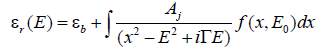(1)

With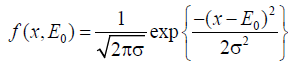(2)

For N oscillator: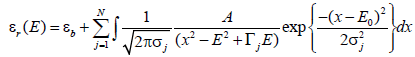(3)

With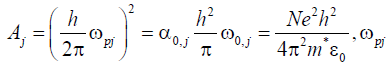Being similar to a plasma pulsation, α0, j polarizability of the exciton, εb the high-frequency dielectric constant of the material (outside the excitonic resonance), Г the spectral width, h the Planck constant, ω0 is the resonance frequency, N the number of particles, e0 the elementary charge electron, m* is the effective mass and x is the resonance energy of excitons.

The inhomogeneous model allows to determine the exciton energy E0,j, the oscillator strength Aj and the parameters for the widening of the excitonic ray (h/2π). Гj, it includes in general the influence of the temperature and the quality of the material (homogeneity, fluctuations in thickness etc.).

Moreover, the modeling of the optical reflectivity, one can do an investigation of the thermal behavior and turbulent of the system with the spectral widening which is a function of the temperature “T” and the effective masse “m*=0.59m0” of the exciton defined by: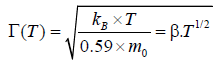With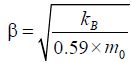(4)

T: Temperature

m0: Mass of a free electron

kB: Constant of Boltzmann.

#### Results and Discussion

Experimentally the optical characterization of the a-plane oriented ZnO shows three types of excitons A, B and C, according to the choice of the polarization, parallel or perpendicular. The energy of the three excitons have been found experimentally by authors  equal to EA=3.398 eV (ωA=0.516 × 1014s-1), EB=3.410 eV (ωB=0.518 × 1014) and Ec=3.438 eV (ωB=0.522 × 1014). Diouf et al.  investigated theoretically and found almost the same values than the experimental values than Lo et al . by using the Gaussian distribution defined by: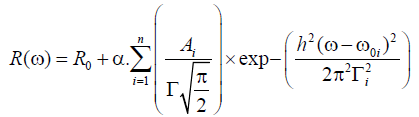(5)

Where the coefficient α is related to the oscillator strength (4πNe02/m*), ω0 is the resonance frequency e0=e/4πε0 with “e” designated as the elementary charge electron R0 the reflection coefficient Ai the spectral area and Γi the spectral width. In the present study one built a program from the inhomogeneous model (1) to investigate the optical properties of the a-plane oriented ZnO. The results obtained by simulation are summarized in the Table 1 compared to the results found experimentally by the authors  (Table 2). A comparison of the tables shows that the inhomogeneous model Figure 1a and Figure 2a, is more accurate than the model used by the authors  to plot the reflectivity curve of the exciton A B. Otherwise one insists on the fact that the parameters summarized in the (Table 1) are used to model the theoretical reflectivity of the exciton A B. In Table 2 one has the values used by the authors  to have their best fit of the free excitons. The method used with the inhomogeneous model allowed furthermore the excitons A B Figure 1b, to plot the free exciton C shown in the Figure 2b thing found by Diouf . One can explain the phenomenon but the fact that the reflectivity curves are composed by several Gaussian shapes thus with the inhomogeneous model according to the Gaussian distribution it was easy to find the reflectivity curves by playing in the value of the oscillator strength for more accurate. As well a comparison between the authors  with the Hopfield model and the inhomogeneous model show important difference summarized in the tables unless the simple Gaussian model (5, used by the authors . One noted that in the range of energy between 3.375 eV and 3.425 eV which represents the reflectivity pic energy range all the models are accurate with the experimental reflectivity curves. But from 3.275 eV to 3.375 eV representing the range of transparency zone one observed that the inhomogeneous model used plots the whole reflectivity curve obtained experimentally by the authors . One can explain this result by the fact that the inhomogeneous model takes accounts the optical properties when the frequencies (ω, are lower than the resonance frequency (ω0, exactly like the simple Gaussian distribution used by authors . The parameters used to plot the free exciton C are summarized in Table 1. The inhomogeneous model can determine separately the both types of widening (Figure 3). The homogeneous widening which depends of the temperature noted Г represents the interaction exciton-photon it increases with the temperature otherwise the inhomogeneous widening depends on the quality of the material through the inhomogeneity of the thickness composition or stress. As well with the inhomogeneous widening it is taken into account through the collection of harmonics oscillators the energy which is distributed according to the Gaussian centered on the average energy E0 and the width halfway up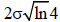.

Free Exciton Frequency (ω0) (s-1) Energy(eV) Oscillator Strength (α, Spectral widening  (Г, (m.s-1 ) Effective mass (m*)
A 5.15E+13
(E=3.390 eV)
1.705 3.25E+12
(Г=2.14 meV,
0.59 m0
B 5.16E+13
(E=3.401 eV)
0.759 4.00E+14
(Г=26.3 meV,
0.59 m0
C 5.21E+13
(E=3.430 eV)
-2,044 2.53E+10
(Г=1.66 meV,
0.59 m0

Table 1: Values of A, B and C free exciton frequency(ω, (s-1), the spatial widening (Г), the effective mass (m*) and the Area (Ai) of the reflective curves obtained by using the Gaussian distribution.

Free Exciton Energy (E) (eV) Oscillator Strength (α, Spectral widening (Г) (meV) Effective mass (m*)
A 3.393 1.708 10.38 0.59 m0
B 3.403 0.77 11.479 0.59 m0

Table 2: Values of A and B free exciton Energy E(eV), Oscillator Strength (α), the spatial widening (Г) and the effective mass (m*) of the exciton obtained by using the Hopfield model .

One used also the spectral widening to investigate the thermal behavior of a-plane oriented ZnO (Figure 4). One noted that from 8 K to 300 K the thermal profile of the sample of ZnO augments with the enhancement of the temperature. One can explain this phenomenon from the relation (4), by the fact that the temperature is an increase function of the spectral widening. Reason why the authors  during their experiments used a cryostat to control the temperature. Unless the others models [28-31] which are limited on the investigation of the c-plane oriented ZnO. But the inhomogeneous model moreover c-plane oriented ZnO allowed to investigate the structure of the a-plane oriented ZnO and one plotted in the same time the excitons A B and C something that one did not find in the literature with the others models unless the model presented recently by the authors [32,33].

#### Conclusion

In this paper one presented another model entitled inhomogeneous model which can be used for the optical characterization. One found the same theoretical reflectivity curves of the free excitons A, B and C in comparison with the experimental results found by Lo et al. by using the same parameters than the authors . Otherwise, one compared the inhomogeneous model with the simple Gaussian distribution used by authors , one has the same results the only difference is the spectral area used in the Gaussian distribution . One investigated as well, the thermal profile of the a-plane oriented ZnO using the spectral widening of the inhomogeneous model. All these results allowed us to present two mathematics models which can be used for the characterization of the nonlinear optical properties with a specific simulation code before getting the experimental results.

#### Acknowledgment

Pr. Bassirou Lo gratefully acknowledges about your help for the experimental results for the investigation of the a-plane oriented ZnO. Pr. Beye gratefully acknowledges for your hospitality in your Optoelectronic Laboratory at the University Cheikh Anta Diop of Dakar.

#### References

Citation: Diouf AA, Lo B, Ngom BD, Beye AC (2018) Inhomogeneous Model for the Investigation of the Optical Properties of the A-Plane Oriented ZnO Epilayers Grown by Plasma-Assisted Molecular Beam Epitaxy. J Laser Opt Photonics 5: 186. DOI: 10.4172/2469-410X.1000186

Copyright: © 2018 Diouf AA, et al. This is an open-access article distributed under the terms of the Creative Commons Attribution License, which permits unrestricted use, distribution, and reproduction in any medium, provided the original author and source are credited.

Select your language of interest to view the total content in your interested language

##### Recommended Journals
Viewmore
###### Article Usage
• Total views: 4613
• [From(publication date): 0-2018 - Nov 17, 2019]
• Breakdown by view type
• HTML page views: 4565Can't read the image? click here to refresh